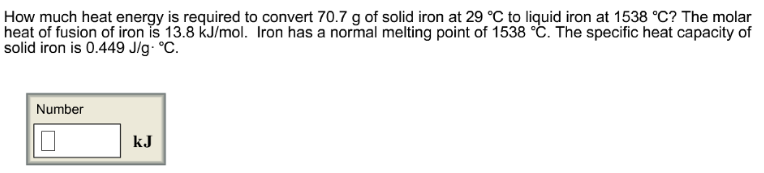# Problem: How much heat energy is required to convert 70.7 g of solid iron at 29 °C to liquid iron at 1538 °C? The molar heat of fusion of iron is 13.8 kJ/mol. Iron has a normal melting point of 1538 °C. The specific heat capacity of solid iron is 0.449 J/g °C.

###### FREE Expert Solution
84% (384 ratings)###### Problem Details

How much heat energy is required to convert 70.7 g of solid iron at 29 °C to liquid iron at 1538 °C? The molar heat of fusion of iron is 13.8 kJ/mol. Iron has a normal melting point of 1538 °C. The specific heat capacity of solid iron is 0.449 J/g °C.Frequently Asked Questions

What scientific concept do you need to know in order to solve this problem?

Our tutors have indicated that to solve this problem you will need to apply the Heating and Cooling Curves concept. You can view video lessons to learn Heating and Cooling Curves. Or if you need more Heating and Cooling Curves practice, you can also practice Heating and Cooling Curves practice problems.

What professor is this problem relevant for?

Based on our data, we think this problem is relevant for Professor Hamann's class at HUNTER.